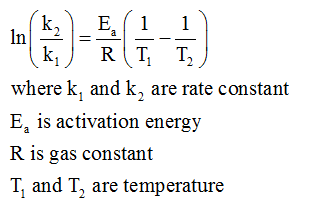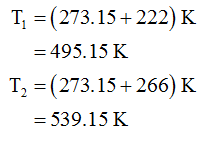# The rate constant of a certain reaction is known to obey the Arrhenius equation, and to have an activation energy Ea = 45.0/kJmol. If the rate constant of this reaction is 1.8 x 104·M−1s−1 at 222.0°C, what will the rate constant be at 266.0°C?

Question
32 views

The rate constant of a certain reaction is known to obey the Arrhenius equation, and to have an activation energy Ea = 45.0/kJmol. If the rate constant of this reaction is 1.8 x 104·M−1s−1 at 222.0°C, what will the rate constant be at 266.0°C?

check_circle

star
star
star
star
star
1 Rating
Step 1

Given information:

Activation energy = 45.0 kJ/mol

Rate constant (k1) = 1.8×104 M-1s-1

Temperature (T1) = 222.0 °C

Temperature (T2) = 266.0 °C

Step 2

Arrhenius equation is represented as follows:Step 3

Since the temperature is given in degree Celsius so it would be converted into Kelvin first as follows:...

### Want to see the full answer?

See Solution

#### Want to see this answer and more?

Solutions are written by subject experts who are available 24/7. Questions are typically answered within 1 hour.*

See Solution
*Response times may vary by subject and question.
Tagged in

### Chemistry